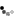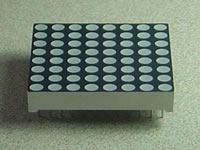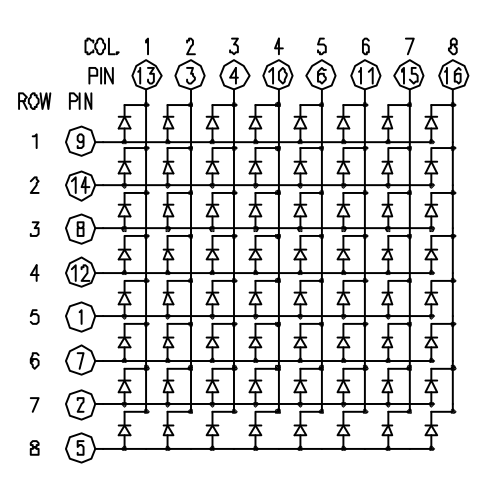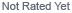Loading... Please wait...

LED Matrix

LED Matrix

LED displays are often packaged as matrixes of LEDs arranged in rows of common anodes and columns of common cathodes, or the reverse. Here's a typical example, and its schematic:These can be very useful displays. To control a matrix, you connect both its rows and columns to your microcontroller. The columns are connected to the LEDs cathodes (see Figure 1), so a column needs to be high for any of the LEDs in that column to turn on. The rows are connected to the LEDs anodes, so the row needs to be low for an individual LED to turn on. If the row and the column are both high or both low, no voltage flows through the LED and it doesn’t turn on.

To control an individual LED, you set its column high and its row low. To control multiple LEDs in a row, you set the rows high, then take the column high, then set the lows row or high as appropriate; a low row will turn the corresponding LED on, and a high row will turn it off.

Although there are pre-made LED matrices, you can also make your own matrix from 64 LEDs, using the schematic as shown above.

It doesn’t matter which pins of the microcontroller you connect the rows and columns to, because you can assign things in software. Connected the pins in a way that makes wiring easiest. A typical layout is shown below.

Here's a matrix of the pin connections, based on the diagram above:

 Matrix pin no. Row Column Arduino pin number 1 5 - 13 2 7 - 12 3 - 2 11 4 - 3 10 5 8 - 16 (analog pin 2) 6 - 5 17 (analog pin 3) 7 6 - 18 (analog pin 4) 8 3 - 19 (analog pin 5) 9 1 - 2 10 - 4 3 11 - 6 4 12 4 - 5 13 - 1 6 14 2 - 7 15 - 7 8 16 - 8 9

Hardware Required

• Arduino Board
• (1) 8 x 8 LED Matrix
• (2) potentiometers
• hook-up wire

Circuit

The 16 pins of the matrix are hooked up to 16 pins of the Arduino. Four of the analog pins are used as digital inputs 16 through 19. The order of the pins is assigned in two arrays in the code.

Two potentiometers, connected to analog pins 0 and 1, control the movement of a lit LED in the matrix.

click the image to enlarge

image developed using Fritzing. For more circuit examples, see the Fritzing project page

Schematic:

click the image to enlarge.

Code

/*
Row-Column Scanning an 8x8 LED matrix with X-Y input

created 27 May 2009
modified 30 Aug 2011
by Tom Igoe

This example works for the Lumex  LDM-24488NI Matrix. See
http://sigma.octopart.com/140413/datasheet/Lumex-LDM-24488NI.pdf
for the pin connections

For other LED cathode column matrixes, you should only need to change
the pin numbers in the row[] and column[] arrays

rows are the anodes
cols are the cathodes
---------

Pin numbers:
Matrix:
* Digital pins 2 through 13,
* analog pins 2 through 5 used as digital 16 through 19
Potentiometers:
* center pins are attached to analog pins 0 and 1, respectively
* side pins attached to +5V and ground, respectively.

This example code is in the public domain.

http://www.arduino.cc/en/Tutorial/RowColumnScanning

*/

// 2-dimensional array of row pin numbers:
const int row = {
2,7,19,5,13,18,12,16 };

// 2-dimensional array of column pin numbers:
const int col = {
6,11,10,3,17,4,8,9  };

// 2-dimensional array of pixels:
int pixels;

// cursor position:
int x = 5;
int y = 5;

void setup() {
// initialize the I/O pins as outputs
// iterate over the pins:
for (int thisPin = 0; thisPin < 8; thisPin++) {
// initialize the output pins:
pinMode(col[thisPin], OUTPUT);
pinMode(row[thisPin], OUTPUT);
// take the col pins (i.e. the cathodes) high to ensure that
// the LEDS are off:
digitalWrite(col[thisPin], HIGH);
}

// initialize the pixel matrix:
for (int x = 0; x < 8; x++) {
for (int y = 0; y < 8; y++) {
pixels[x][y] = HIGH;
}
}
}

void loop() {

// draw the screen:
refreshScreen();
}

// turn off the last position:
pixels[x][y] = HIGH;
// read the sensors for X and Y values:
x = 7 - map(analogRead(A0), 0, 1023, 0, 7);
y = map(analogRead(A1), 0, 1023, 0, 7);
// set the new pixel position low so that the LED will turn on
// in the next screen refresh:
pixels[x][y] = LOW;

}

void refreshScreen() {
// iterate over the rows (anodes):
for (int thisRow = 0; thisRow < 8; thisRow++) {
// take the row pin (anode) high:
digitalWrite(row[thisRow], HIGH);
// iterate over the cols (cathodes):
for (int thisCol = 0; thisCol < 8; thisCol++) {
// get the state of the current pixel;
int thisPixel = pixels[thisRow][thisCol];
// when the row is HIGH and the col is LOW,
// the LED where they meet turns on:
digitalWrite(col[thisCol], thisPixel);
// turn the pixel off:
if (thisPixel == LOW) {
digitalWrite(col[thisCol], HIGH);
}
}
// take the row pin low to turn off the whole row:
digitalWrite(row[thisRow], LOW);
}
}

There is a more complex example in the Arduino playground. Other examples can be found on Tom Igoe's blog:

New Products

•••••What's News

• Using a Generic STM32 board with Arduino

Using a Generic STM32 Board with ArduinoIf you haven't got one already, you can …

• Using a SIM800l GSM/GPRS Cellphone Module to send an SMS message

This example shows how to use a low-cost SIM800l GSM/GPS Module to send an SMS …

• Using MQTT with ESP8266

This blog entry is quick outline of using MQTT with a message broker to …